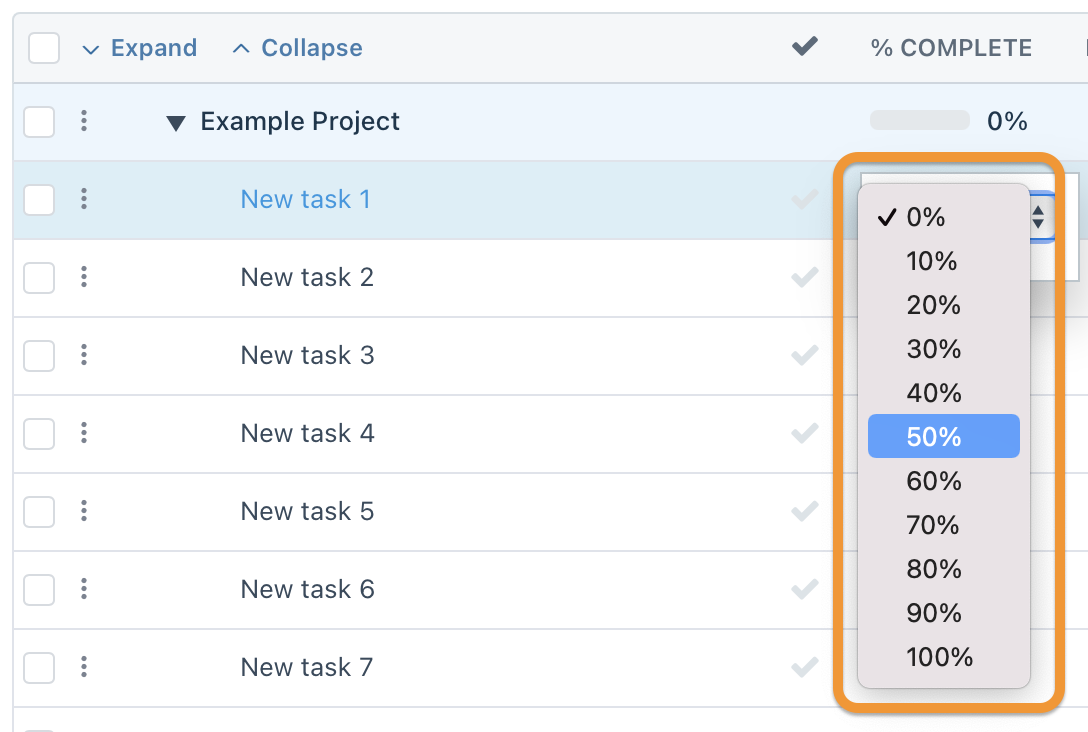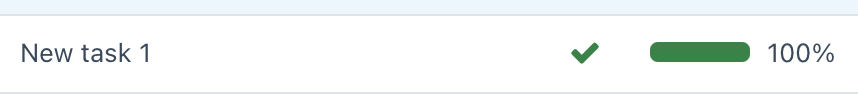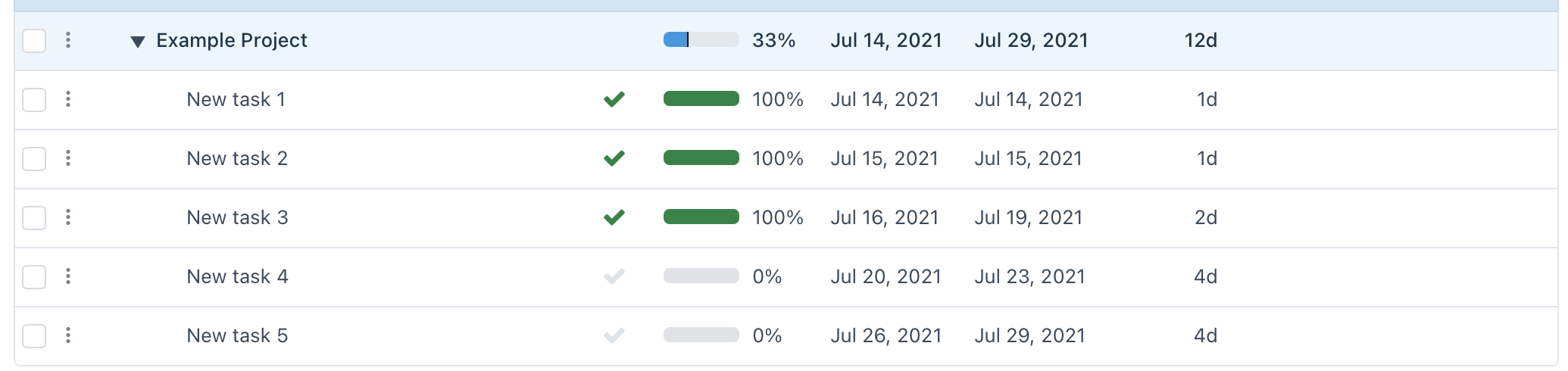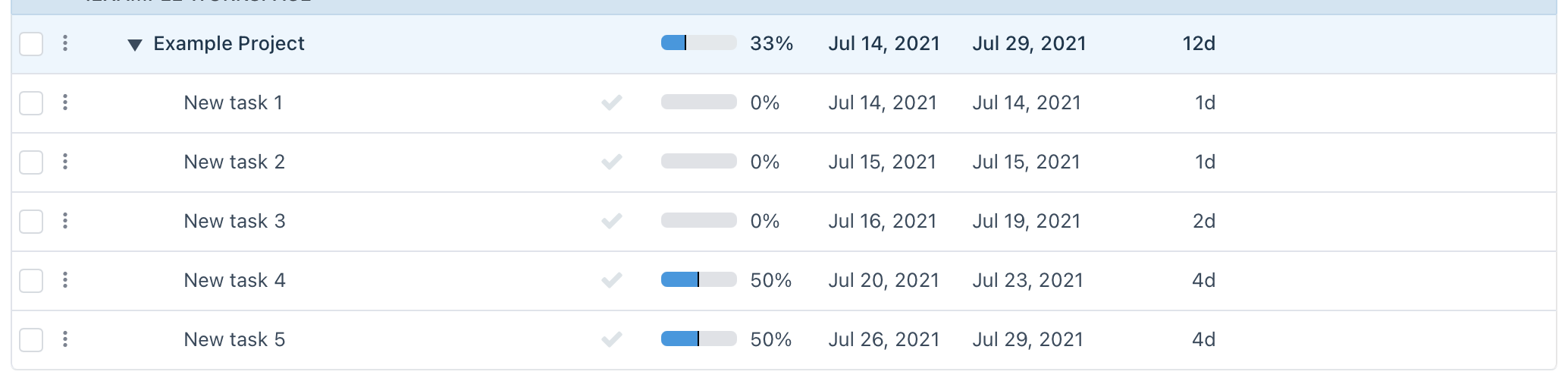# % Complete

Workzone calculates a percent value for both summary tasks and subtasks, as well as projects. This value can be useful to determine progress at a glance. The percent value is displayed in the "% COMPLETE" column in various views and reports.

At the lowest task level, the percent value can be adjusted in ten percent increments (10%, 20%, 30%, etc.) by clicking on the value currently displayed in the row's "% COMPLETE" column and choosing the appropriate value from the drop down, as seen here:Also, a task row's percentage can be set to 100% by marking the task as complete from reports (such as the To-Do List) or the checkmark symbol column ( ✓ ).# Project Calculation

At the top level, projects in Workzone do not provide any means of manually setting the % Complete value. Rather, the % Complete value of a project is directly determined by its subtasks and their % Complete (summary tasks follow this same behavior), as it relates to the value found in the WORK column.

NOTE: If the WORK column isn't displayed, the DURATION column value will provide the same function described above. Check out Work vs. Duration and Dates, Durations, and Milestones for more information.

Here are a couple of projects with multiple tasks in various states of completion to provide some examples:In the above depicted project, two of the tasks are incomplete, while the rest are completed. The total length of the project is 12 days, with the completed tasks adding up to four days (1 + 1 + 2), with the remainder incomplete. Four out of twelve days completed is rounded to 33%.

Here's a different example:Here, you can see that 50% of each of the 4 day tasks are marked complete, which calculates to four days of completion (50% of 4 = 2, and 2 + 2 = 4) out of the twelve, which is also rounded to 33%.